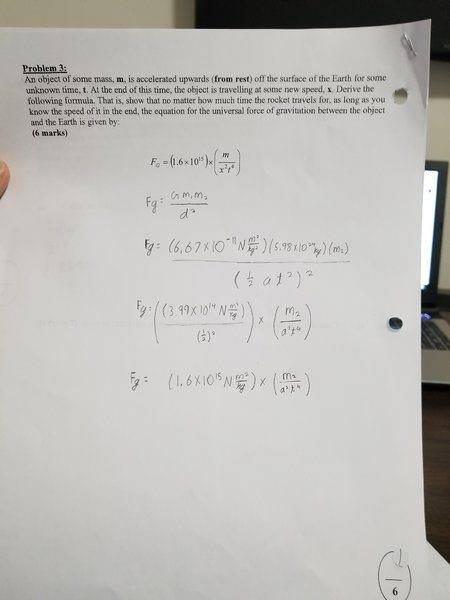# An object of some mass, m, is accelerated upwards....

• Vraj Patel
In summary, the conversation discusses a problem involving an object being accelerated upwards from the surface of the Earth and deriving a formula for the universal force of gravitation between the object and the Earth. It is noted that the gravitational force depends on the distance to the center of the Earth and not just the distance above the surface. After some discussion and clarification, it is discovered that the original formula given in the problem was incorrect due to a typo.

## Homework Statement

an object of some mass, m, is accelerated upwards (from rest) off the surface off the Earth for some unknown time, t. At the end of this time, the object is traveling at some new speed, x. Derive the following formula. That is, show that no matter how much time the rocket travels for, as long as you know the speed of it in the end, the equation for the universal force of gravitation between the object and the Earth is given by:
FG = (1.6x10^15) x (m/x^2t^4)

## Homework Equations

FG = (1.6x10^15) x (m/x^2t^4)

## The Attempt at a Solution

I have attached a photo of my work, to the point that I got stuck on.#### Attachments

This problem doesn't quite make sense to me. Where did you get it?

(Note that the gravitational force depends on the distance to the center of the earth, not just on the distance above the surface.)

Doc Al said:
This problem doesn't quite make sense to me. Where did you get it?

(Note that the gravitational force depends on the distance to the center of the earth, not just on the distance above the surface.)

This is question I have been stuck on, in my weekly assignment. May you explain the part of the problem that doesn't make sense.

Vraj Patel said:
May you explain the part of the problem that doesn't make sense.
Plug in a few values for speed and time. If the distance traveled in that time is small, the gravitational force should equal mg. Does it?

Doc Al said:
Plug in a few values for speed and time. If the distance traveled in that time is small, the gravitational force should equal mg. Does it?

would you like me to plug it into the FG = (1.6x10^15) x (m/x^2t^4) formula.

Vraj Patel said:
would you like me to plug it into the FG = (1.6x10^15) x (m/x^2t^4) formula.
Yes.

Doc Al said:
Yes.
I am sorry for using your time, but my teacher made a typo, and I figured out the problem.

Vraj Patel said:
I am sorry for using your time, but my teacher made a typo, and I figured out the problem.
That's ok - it happens. (Hope I am not being presumptuous answering for Doc Al.)

Vraj Patel said:
I am sorry for using your time, but my teacher made a typo, and I figured out the problem.
No problem at all. I was leading you to plug in some values that would convince you (and your teacher!) that the given formula cannot possibly be correct. But no harm done. I'm glad your teacher found the typo.

haruspex said:
(Hope I am not being presumptuous answering for Doc Al.)
Not at all.I agree with you.